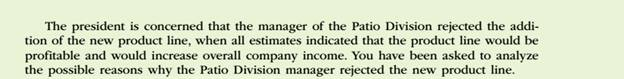Chapter
Section
Textbook ProblemTo determine

Concept Introduction:

Return on investment (ROI):

Return on investment is a profitability ratio that represents the percentage return on the investment made. It is calculated by dividing the Net Income by the Average total assets. The formulas to calculate the ROI are as follows:

ROI = Operating IncomeAverage total assets

ROI (Expanded)= Operating IncomeSales ×SalesAverage total assets

Or

ROI = Profit Margin Ratio ×Asset Turnover ratio

To Calculate:

The estimated return on investment after adding the new product line

Explanation

The estimated return on investment after adding the new product line is calculated as follows:

 Operating Income (A) (3600000+810000) \$ 4,410,000

Still sussing out bartleby?

Check out a sample textbook solution.

See a sample solution

The Solution to Your Study Problems

Bartleby provides explanations to thousands of textbook problems written by our experts, many with advanced degrees!

Get Started

Discuss the importance of complementary effects in a keep-or-drop decision.

Managerial Accounting: The Cornerstone of Business Decision-Making

How do flexible benefit plans work?

Foundations of Business (MindTap Course List)

PRESENT VALUE OF AN ANNUITY Find the present values of these ordinary annuities. Discounting occurs once 4 year...

Fundamentals of Financial Management, Concise Edition (with Thomson ONE - Business School Edition, 1 term (6 months) Printed Access Card) (MindTap Course List)